|

# 诺贝尔瓷砖钟树铭:四重升级,打造全球新一代瓷砖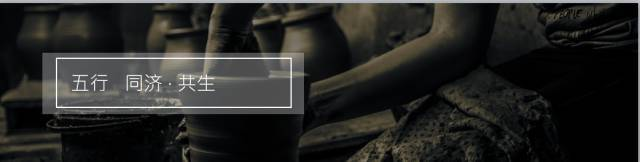China的含义之一是陶瓷。中国人懂陶瓷，懂得欣赏陶瓷，也喜欢陶瓷，更愿意去亲近陶瓷。瓷砖作为陶瓷的重要品类，自古以来就是皇室贵族才能享受的奢侈品。直到1930年代，全中国用得起瓷砖的也只有一户人家，就在南京的总统府。大家想了解当时的情景，只要买张参观门票就可以看到了。

改革开放之后瓷砖进入了我们的生活，承载了现代生活的居住空间。

对一片好的瓷砖人们提出各式各样的要求。我们诺贝尔人本着对瓷砖的理解与追求，不断地探索、创造更好的瓷砖。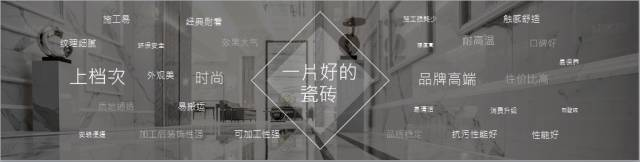我们结合了来自三个国家、五大研发机构的力量，300人的独立项目研发队伍， 18万个日日夜夜，更有那数不清道不尽的汗水、泪水，最终集结而成了独有SIMM-TEC核心技术的瓷抛砖。

SIMM-TEC四大核心技术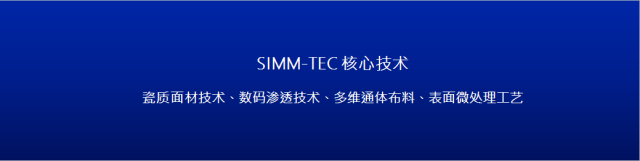这里展示一些形象的图片，便于大家了解SIMM-TEC核心技术。

技术一

●以新型瓷面替代传统釉面 ●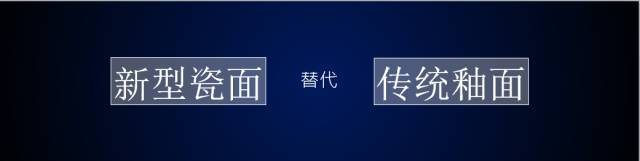釉是附着在陶瓷表面的玻璃层，简单说，釉其实是一种玻璃的物质，而瓷是以晶相结构为主的物质。这张图可以帮助大家形象地理解玻璃与陶瓷的差异性，一个是温润、饱满、厚实，另外一个是晶亮、剔透、轻莹。诺贝尔瓷抛砖走的是这条温润、饱满而厚实的瓷质面材道路，其优越性是表面更耐磨，触感更温润。

技术二

●以立体渗花替代平面印花●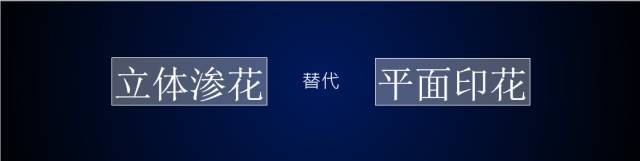我们透过喷墨技术将离子团渗入瓷质表层，使之与表层的特殊材料产生新的作用，进而产生新的组合发出颜色。所以，这是由里而外的发色技术。

我用通俗的比喻帮助大家理解这个技术：首先，传统的印刷方式不论男女老少，高矮黑白，先打一层厚粉底。就像我今天上台时对化妆的小弟说，我不太习惯这么厚的粉底，可不可以打薄一点？但他说，你的皮肤有点黑，要把粉底打得厚一点一样的道理。我们一层一层补妆上去，最后用一层玻璃覆盖住整个妆容，结果就一目了然。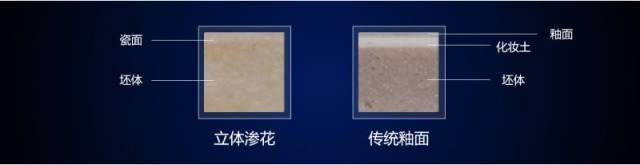这张图是瓷砖的断面图，可以看得十分明显，立体渗花是由里到外展现，呈现出几乎难以辨别的一致性；而传统釉面，像个三明治，可以看出明显的分层。我们可以这样打个比方，立体渗花带来的优势，首先是离子级的精度，而不是像素，以及“不打粉底”，“不施釉”，通体内外一致的发色原理，花纹当然就更逼真了。

技术三

●以多维通体布料替代一次布料●相信在座的各位都能选出下列图片中哪两片是通体布料；如果还是选不出来，再看这张图。由里到外通体一致，再加上我们适当的将坯体增厚的技术，产生由里到外一致的立体加工线条，瓷抛砖可以说开创了瓷砖的新风貌。所以说，多维通体布料带来的就是质感更通体，质地也更厚实。

技术四

●微米级表面处理技术替代传统硬抛光技术●抛光砖要抛去面层大概1MM的厚度，新型的微处理工艺只要抛去0.1MM，大大减低了整个环境的压力，而且抛过的废料还可以再回收利用，这是一种节能、环保、安全的材料。

一个技术的成熟不是单一的，必须靠相关环节紧紧相扣，缺一个环节都影响整体呈现。

以上技术体现出瓷抛砖的五大优势

首先表面更耐磨

第二花纹更逼真

第三质地更通体

第四质感更厚实

第五触感更温润

以科技师法自然，以设计重塑本真

接下来，我们谈一下产品开发的核心理念。

大家会问，为什么一款产品花五年时间才做出来？这是因为，瓷抛砖的研发工艺的难度在全球也是绝无仅有的。首先，每一片瓷抛砖里面的每一道工艺，每一道工艺背后的发色原理，都是难关，只要是一个难关就是世界级的难关。为什么要三个国家来协助呢，因为这些课题都是世界级的课题，它们隐藏在每一道工艺和每一道发色里面，解决一片砖基本上就完成了瓷砖的一次科研飞跃。

以科技师法自然，以设计重塑本真——这个核心的理念并非一句口号。因为瓷抛砖并非存在于想象中，它需要以技术的参与，将实物创造出来。以科技师法自然，以设计重塑本真，难在重塑本真。因为“本真”不能打折扣，这就需要真正的科技研发精神。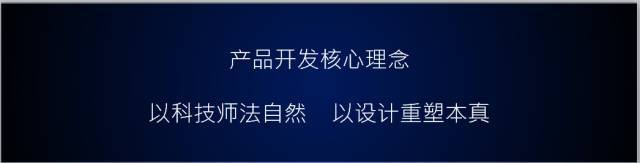我们凭借这个核心理念，开发出一系列产品，目前有34款花色，60款产品。大家可以看到，色系从白到米白，黄色到米黄色，到金色，深金，浅金，直到冷色调，暖色调，灰色的深棕浅，到深灰色，深黑色，特殊色，及金色，顶级的绿色，纯蓝色，紫萝红（一种暗红色），再加上黑色与金线结合的黑金花……为什么我要强调色系，因为瓷抛砖的调色相当困难。我们的全系列产品获得了中国建材联合会所有权威专家一致首肯，认为瓷抛砖的整体技术处于世界领先水平。

我举几个例子做进一步解释。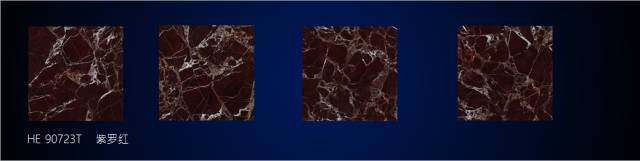这一片叫紫罗红。我对它的感情非常深，因为8个月搞一片砖，奋斗的时间非常长。有人问瓷抛砖的研发为什么这么难，我打个简单比方：一杯浓稠的牛奶跟一杯开水同时调这样深的色调，可能浓稠的牛奶根本调不出来。我可以很负责任地说，这四片砖我们仅在颜色处理就用了64道工序。我从事陶瓷将近30年，没有遇过这么复杂的产品，这是空前的，我希望最好也不要再有，因为实在太难了。

黑白是最经典的颜色。请大家注意这白色的砖，它的通体性最能看出该企业选材的标准。你要做得白，选的料一定要好；再看黑色，要把最黑的饱和度通过原料做出来是相当难的。市场上黑色的仿石瓷砖产品很多，但都达不到这个效果。近看鱼肚白，用料纯净洁白，饱满有微透感，质地厚实，触感温润细致，能带来沁人心脾的静谧感。

先看这个白色的砖，我们俗称鱼肚白，近看它会发现白坯中缓缓透析到面层的淡蓝色，具有汉白玉一般的透感。触摸它的时候会感到质地非常细致，能给人一种沁人心脾的静谧感。再远观这块白色砖的纹理，分布自然，磅礴大气，每一个层次的绽放，给人以畅快之感。请允许我吟一句诗词以表达我对这块砖的感情:“雨过天青云破处，这般颜色做将来”。图中这款黑金花的实际取材者就在现场，他是意大利合作伙伴的首席设计师。黑金花，顾名思义就是坯体要黑，线条要金，这样的品相最上乘。不过，即使很次的线条，也已是罕见与稀有，因为这样的石材已经买不到了。近看它颜色饱满深沉，由里到外一黑到底，黑得干脆，黑得畅快。我们再远观，它的纹理雄浑有力，还可以看到细节——一种暗裂纹的处理细致刚毅，可以说将这款黑色石材中的王者表现得淋漓尽致，它的工艺与表现力绝对经得起像尹博士这样专家级的严苛眼光。

我们可以这样说：瓷抛砖的每一片砖，每一个工艺、每一个环节都蕴藏了诺贝尔人对陶瓷的虔诚工匠心。因为陶瓷是非常老实的，你对它做了什么它一定会反映出来。这是我上学时老师讲的第一句话。陶瓷是很诚实的东西，你不能骗它。除了工匠心，瓷抛砖还蕴含了诺贝尔人的科技精神。每一个年代创造的陶瓷新历史都是由科技产生的，我们这一代也是如此。瓷砖的最后一里路大家都知道——一片瓷砖最终还是要在用户家里得到很好的展示才是最重要的。在座的很多设计师都是我的前辈，比我还具有发言权。我们相信瓷抛砖的核心技术及其五大优势，一定能给设计师拓展更广阔的设计空间，同时带来更优质的选择。最后，我用一个设计师作品与大家分享瓷抛砖应用中的美感。我们看这个大平层用了五片砖，入门的银雕灰,切槽的处理体现出门头的气派，具有立体感；两侧使用的黑金花沉稳而庄重；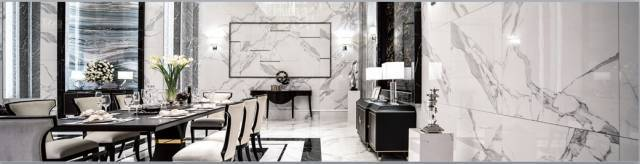墙壁一体用了鱼肚白，以及现代感的黑色砖；最后正中的背景墙用了蔚蓝海岸的背景墙，有一种流动的韵律感。

这些精心设计充分体现出诺贝尔瓷抛砖在应用中的大气、庄重、现代、经典，呈给我们以十分的想象空间。

各位朋友，诺贝尔五年磨一剑！瓷抛砖以独有的SIMM-TEC核心技术实现中国瓷砖一次新的科技飞跃，开创了中国建筑陶瓷的新篇章，感谢各位来宾、各位朋友的共同见证！

`声明：本文由入驻焦点开放平台的作者撰写，除焦点官方账号外，观点仅代表作者本人，不代表焦点立场错误信息举报电话： 400-099-0099，邮箱：jubao@vip.sohu.com，或点此进行意见反馈，或点此进行举报投诉。`A B C D E F G H J K L M N P Q R S T W X Y Z
A - B - C - D - E
• A
• 鞍山
• 安庆
• 安阳
• 安顺
• 安康
• 澳门
• B
• 北京
• 保定
• 包头
• 巴彦淖尔
• 本溪
• 蚌埠
• 亳州
• 滨州
• 北海
• 百色
• 巴中
• 毕节
• 保山
• 宝鸡
• 白银
• 巴州
• C
• 承德
• 沧州
• 长治
• 赤峰
• 朝阳
• 长春
• 常州
• 滁州
• 池州
• 长沙
• 常德
• 郴州
• 潮州
• 崇左
• 重庆
• 成都
• 楚雄
• 昌都
• 慈溪
• 常熟
• D
• 大同
• 大连
• 丹东
• 大庆
• 东营
• 德州
• 东莞
• 德阳
• 达州
• 大理
• 德宏
• 定西
• 儋州
• 东平
• E
• 鄂尔多斯
• 鄂州
• 恩施
F - G - H - I - J
• F
• 抚顺
• 阜新
• 阜阳
• 福州
• 抚州
• 佛山
• 防城港
• G
• 赣州
• 广州
• 桂林
• 贵港
• 广元
• 广安
• 贵阳
• 固原
• H
• 邯郸
• 衡水
• 呼和浩特
• 呼伦贝尔
• 葫芦岛
• 哈尔滨
• 黑河
• 淮安
• 杭州
• 湖州
• 合肥
• 淮南
• 淮北
• 黄山
• 菏泽
• 鹤壁
• 黄石
• 黄冈
• 衡阳
• 怀化
• 惠州
• 河源
• 贺州
• 河池
• 海口
• 红河
• 汉中
• 海东
• 怀来
• I
• J
• 晋中
• 锦州
• 吉林
• 鸡西
• 佳木斯
• 嘉兴
• 金华
• 景德镇
• 九江
• 吉安
• 济南
• 济宁
• 焦作
• 荆门
• 荆州
• 江门
• 揭阳
• 金昌
• 酒泉
• 嘉峪关
K - L - M - N - P
• K
• 开封
• 昆明
• 昆山
• L
• 廊坊
• 临汾
• 辽阳
• 连云港
• 丽水
• 六安
• 龙岩
• 莱芜
• 临沂
• 聊城
• 洛阳
• 漯河
• 娄底
• 柳州
• 来宾
• 泸州
• 乐山
• 六盘水
• 丽江
• 临沧
• 拉萨
• 林芝
• 兰州
• 陇南
• M
• 牡丹江
• 马鞍山
• 茂名
• 梅州
• 绵阳
• 眉山
• N
• 南京
• 南通
• 宁波
• 南平
• 宁德
• 南昌
• 南阳
• 南宁
• 内江
• 南充
• P
• 盘锦
• 莆田
• 平顶山
• 濮阳
• 攀枝花
• 普洱
• 平凉
Q - R - S - T - W
• Q
• 秦皇岛
• 齐齐哈尔
• 衢州
• 泉州
• 青岛
• 清远
• 钦州
• 黔南
• 曲靖
• 庆阳
• R
• 日照
• 日喀则
• S
• 石家庄
• 沈阳
• 双鸭山
• 绥化
• 上海
• 苏州
• 宿迁
• 绍兴
• 宿州
• 三明
• 上饶
• 三门峡
• 商丘
• 十堰
• 随州
• 邵阳
• 韶关
• 深圳
• 汕头
• 汕尾
• 三亚
• 三沙
• 遂宁
• 山南
• 商洛
• 石嘴山
• T
• 天津
• 唐山
• 太原
• 通辽
• 铁岭
• 泰州
• 台州
• 铜陵
• 泰安
• 铜仁
• 铜川
• 天水
• 天门
• W
• 乌海
• 乌兰察布
• 无锡
• 温州
• 芜湖
• 潍坊
• 威海
• 武汉
• 梧州
• 渭南
• 武威
• 吴忠
• 乌鲁木齐
X - Y - Z
• X
• 邢台
• 徐州
• 宣城
• 厦门
• 新乡
• 许昌
• 信阳
• 襄阳
• 孝感
• 咸宁
• 湘潭
• 湘西
• 西双版纳
• 西安
• 咸阳
• 西宁
• 仙桃
• 西昌
• Y
• 运城
• 营口
• 盐城
• 扬州
• 鹰潭
• 宜春
• 烟台
• 宜昌
• 岳阳
• 益阳
• 永州
• 阳江
• 云浮
• 玉林
• 宜宾
• 雅安
• 玉溪
• 延安
• 榆林
• 银川
• Z
• 张家口
• 镇江
• 舟山
• 漳州
• 淄博
• 枣庄
• 郑州
• 周口
• 驻马店
• 株洲
• 张家界
• 珠海
• 湛江
• 肇庆
• 中山
• 自贡
• 资阳
• 遵义
• 昭通
• 张掖
• 中卫

1室1厅1厨1卫1阳台

1
2
3
4
5

0
1
2

1

1

0
1
2
3报名成功，资料已提交审核A B C D E F G H J K L M N P Q R S T W X Y Z
A - B - C - D - E
• A
• 鞍山
• 安庆
• 安阳
• 安顺
• 安康
• 澳门
• B
• 北京
• 保定
• 包头
• 巴彦淖尔
• 本溪
• 蚌埠
• 亳州
• 滨州
• 北海
• 百色
• 巴中
• 毕节
• 保山
• 宝鸡
• 白银
• 巴州
• C
• 承德
• 沧州
• 长治
• 赤峰
• 朝阳
• 长春
• 常州
• 滁州
• 池州
• 长沙
• 常德
• 郴州
• 潮州
• 崇左
• 重庆
• 成都
• 楚雄
• 昌都
• 慈溪
• 常熟
• D
• 大同
• 大连
• 丹东
• 大庆
• 东营
• 德州
• 东莞
• 德阳
• 达州
• 大理
• 德宏
• 定西
• 儋州
• 东平
• E
• 鄂尔多斯
• 鄂州
• 恩施
F - G - H - I - J
• F
• 抚顺
• 阜新
• 阜阳
• 福州
• 抚州
• 佛山
• 防城港
• G
• 赣州
• 广州
• 桂林
• 贵港
• 广元
• 广安
• 贵阳
• 固原
• H
• 邯郸
• 衡水
• 呼和浩特
• 呼伦贝尔
• 葫芦岛
• 哈尔滨
• 黑河
• 淮安
• 杭州
• 湖州
• 合肥
• 淮南
• 淮北
• 黄山
• 菏泽
• 鹤壁
• 黄石
• 黄冈
• 衡阳
• 怀化
• 惠州
• 河源
• 贺州
• 河池
• 海口
• 红河
• 汉中
• 海东
• 怀来
• I
• J
• 晋中
• 锦州
• 吉林
• 鸡西
• 佳木斯
• 嘉兴
• 金华
• 景德镇
• 九江
• 吉安
• 济南
• 济宁
• 焦作
• 荆门
• 荆州
• 江门
• 揭阳
• 金昌
• 酒泉
• 嘉峪关
K - L - M - N - P
• K
• 开封
• 昆明
• 昆山
• L
• 廊坊
• 临汾
• 辽阳
• 连云港
• 丽水
• 六安
• 龙岩
• 莱芜
• 临沂
• 聊城
• 洛阳
• 漯河
• 娄底
• 柳州
• 来宾
• 泸州
• 乐山
• 六盘水
• 丽江
• 临沧
• 拉萨
• 林芝
• 兰州
• 陇南
• M
• 牡丹江
• 马鞍山
• 茂名
• 梅州
• 绵阳
• 眉山
• N
• 南京
• 南通
• 宁波
• 南平
• 宁德
• 南昌
• 南阳
• 南宁
• 内江
• 南充
• P
• 盘锦
• 莆田
• 平顶山
• 濮阳
• 攀枝花
• 普洱
• 平凉
Q - R - S - T - W
• Q
• 秦皇岛
• 齐齐哈尔
• 衢州
• 泉州
• 青岛
• 清远
• 钦州
• 黔南
• 曲靖
• 庆阳
• R
• 日照
• 日喀则
• S
• 石家庄
• 沈阳
• 双鸭山
• 绥化
• 上海
• 苏州
• 宿迁
• 绍兴
• 宿州
• 三明
• 上饶
• 三门峡
• 商丘
• 十堰
• 随州
• 邵阳
• 韶关
• 深圳
• 汕头
• 汕尾
• 三亚
• 三沙
• 遂宁
• 山南
• 商洛
• 石嘴山
• T
• 天津
• 唐山
• 太原
• 通辽
• 铁岭
• 泰州
• 台州
• 铜陵
• 泰安
• 铜仁
• 铜川
• 天水
• 天门
• W
• 乌海
• 乌兰察布
• 无锡
• 温州
• 芜湖
• 潍坊
• 威海
• 武汉
• 梧州
• 渭南
• 武威
• 吴忠
• 乌鲁木齐
X - Y - Z
• X
• 邢台
• 徐州
• 宣城
• 厦门
• 新乡
• 许昌
• 信阳
• 襄阳
• 孝感
• 咸宁
• 湘潭
• 湘西
• 西双版纳
• 西安
• 咸阳
• 西宁
• 仙桃
• 西昌
• Y
• 运城
• 营口
• 盐城
• 扬州
• 鹰潭
• 宜春
• 烟台
• 宜昌
• 岳阳
• 益阳
• 永州
• 阳江
• 云浮
• 玉林
• 宜宾
• 雅安
• 玉溪
• 延安
• 榆林
• 银川
• Z
• 张家口
• 镇江
• 舟山
• 漳州
• 淄博
• 枣庄
• 郑州
• 周口
• 驻马店
• 株洲
• 张家界
• 珠海
• 湛江
• 肇庆
• 中山
• 自贡
• 资阳
• 遵义
• 昭通
• 张掖
• 中卫• 手机• 分享
• 设计
免费设计
• 计算器
装修计算器
• 入驻
合作入驻
• 联系
联系我们
• 置顶
返回顶部